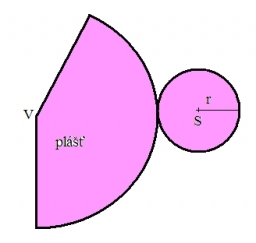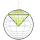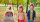# Surface and volume

Find the surface and volume of the rotating cone if the circumference of its base is 62.8 m and the side is 25 m long.

S =  1098.8408 m2
V =  2397.2302 m3

### Step-by-step explanation:Did you find an error or inaccuracy? Feel free to write us. Thank you!Tips to related online calculators
Pythagorean theorem is the base for the right triangle calculator.
Tip: Our volume units converter will help you with the conversion of volume units.

#### You need to know the following knowledge to solve this word math problem:

We encourage you to watch this tutorial video on this math problem:

## Related math problems and questions:

• Rotating coneFind the rotating cone's surface and volume if its side is 150 mm long and the circumference of the base is 43.96 cm.
• Cone - sideFind the cone's surface area and volume if its height is 125 mm and the side length is 17 cm.
• The rotatingThe rotating cone has a height of 0.9 m and the diameter of the base is 7.2 dm. Calculate the surface of the cone. (Hint: use Pythagorean theorem for a side of cone)
• Rotating coneCalculate the volume and the surface area of a rotating cone of base radius r = 2.3 dm and a height h = 46 mm.
• Cone - from volume surface areaThe volume of the rotating cone is 1,018.87 dm3, and its height is 120 cm. What is the surface area of the cone?
• The cone - S,VCalculate the volume and surface area of the cone if its radius r = 6 cm and side s = 10 cm.
• Angle of deviationThe surface of the rotating cone is 30 cm2 (with circle base), its surface area is 20 cm2. Calculate the deviation of the side of this cone from the plane of the base.
• PrismFind the volume and surface area of prism with base of an equilateral triangle with side 7 dm long and the body height of 1.5 m.
• ConeCalculate the volume of the rotating cone with a base radius 26.3 cm and a side 38.4 cm long.
• Cone area and sideCalculate the surface area and volume of a rotating cone with a height of 1.25 dm and 17,8dm side.
• The Indian tentThe Indian tent is cone-shaped. Its height is 3.5 m. The diameter of the base is 2.5 m. How much canvas is needed to make a tire?
• Surface of the coneCalculate the surface of the cone if its height is 8 cm and the volume is 301.44 cm3.
• Conical areaA right angled triangle has sides a=12 and b=19 in right angle. The hypotenuse is c. If the triangle rotates on the c side as axis, find the volume and surface area of conical area created by this rotation.
• Slant heightThe cone's slant height is 5cm, and the radius of its base is 3cm, find the volume of the cone.
• ConeCalculate volume and surface area of ​​the cone with a diameter of the base d=15 cm and side of the cone with the base has angle 52°.
• Lateral surface areaThe ratio of the area of the base of the rotary cone to its lateral surface area is 3: 5. Calculate the surface and volume of the cone, if its height v = 4 cm.
• Rotary coneRotary cone whose height is equal to the circumference of the base, has a volume 229 cm3. Calculate the radius of the base circle and height of the cone.